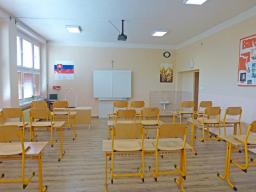# Distinction 2759

There are 500 pupils in the school. At the end of the school year, the principal announced that 20% of the students had received the award. At the same time, 18% of boys and 23% of girls achieved the distinction. Determine how many boys and how many girls attend the school.

x =  300
y =  200

### Step-by-step explanation:

x+y = 500
0.18x+0.23y = 0.2·500

x+y = 500
0.18·x+0.23·y = 0.2·500

x+y = 500
0.18x+0.23y = 100

Row 2 - 0.18000000000001 · Row 1 → Row 2
x+y = 500
0.05y = 10

y = 10/0.05 = 200
x = 500-y = 500-200 = 300

x = 300
y = 200

Our linear equations calculator calculates it.Did you find an error or inaccuracy? Feel free to write us. Thank you!

Tips for related online calculators